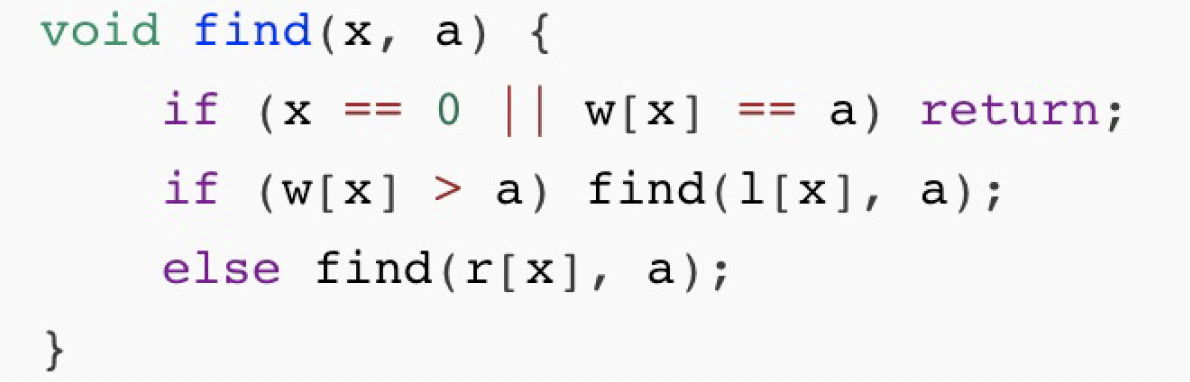시간 제한 메모리 제한 제출 정답 맞은 사람 정답 비율
2 초 256 MB 1 0 0 0.000%

## 문제

This problem is about Binary Search Trees (BST), a basic data structure. The structure is a rooted binary tree which stores values in its nodes. If node $x$ contains value $a$, all values in the left subtree of $x$ are less than $a$, and all values in the right subtree of $x$ are greater than $a$.

In order to unify the details, we provide an implementation of finding a value $a$ in a BST rooted at node $x$:Here, $l[x]$ is the left child of $x$, $r[x]$ is the right child of $x$, and $w[x]$ is the value of $x$. Specifically, if $x$ does not have a left child (right child), $l[x]$ ($r[x]$) is $0$.

We define $A(\mathit{root}, a)$ as the array of all nodes visited by $\text{find}(\mathit{root}, a)$. We also define the cost of $\mathrm{find}(\mathit{root}, a)$ as $$\sum_{v \in A(\mathit{root}, a)} w[v]\text{.}$$

Now there are $n$ empty BSTs and $m$ operations. Your task is to process these operations quickly. There are two different kinds of operations:

• "1 $l$ $r$ $w$". For each $i \in [l, r]$, insert an integer $w$ into the $i$-th BST. It is guaranteed that $w$ is not present in these BSTs. Insertion starts at the root, goes the same as $\mathrm{find}$, but instead of making the last $\mathrm{find}(0, w)$ call, creates a new node with value $w$ there and returns.
• "2 $x$ $a$". Calculate the cost of finding $a$ in the $x$-th BST.

## 입력

The first line contains two integers, $n$ and $m$ ($1 \le n, m \le 2 \cdot 10^{5})$, indicating the number of BSTs and the number of operations.

Then $m$ lines follow. Each line contains description of an operation and is formatted as either "1 $l$ $r$ $w$" ($1 \le l \le r \le n$; $1 \le w \le 10^9$) or "2 $x$ $a$" ($1 \le x \le n$; $1 \le a \le 10^9$).

It is guaranteed that all inserted numbers ($w$ in operations of the first kind) are different from each other.

## 출력

For each operation of the second kind, output a single line with a single integer: the cost.

## 예제 입력 1

3 9
1 1 2 2
1 1 3 1
1 2 3 3
2 1 2
2 1 4
2 2 2
2 2 4
2 3 2
2 3 4


## 예제 출력 1

2
2
2
5
4
4